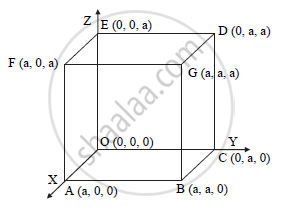# Show that the angle between any two diagonals of a cube is cos^-1(1/3) - Mathematics and Statistics

Show that the angle between any two diagonals of a cube is cos^-1(1/3)

#### SolutionLet ‘a’ be the edge of the cube with one vertex at the origin.
The diagonals of the cube are OG, AD, CF and EB.
Consider the diagonals OG and AD.
The d.r.s of OG are
a - 0, a - 0, a - 0 = a, a, a
0 - a, a -0, a -0 = a, a, a 
Let θ be the angle between OG and AD

costheta=(a(-a)+a(a)+a(a))/(sqrt(a^2+a^2+a^2).sqrt((-a)^2+a^2+a^2))

=a^2/(3a^2)

costheta=1/3

theta=cos^-1(1/3)

Concept: Angle Between Line and a Plane
Is there an error in this question or solution?